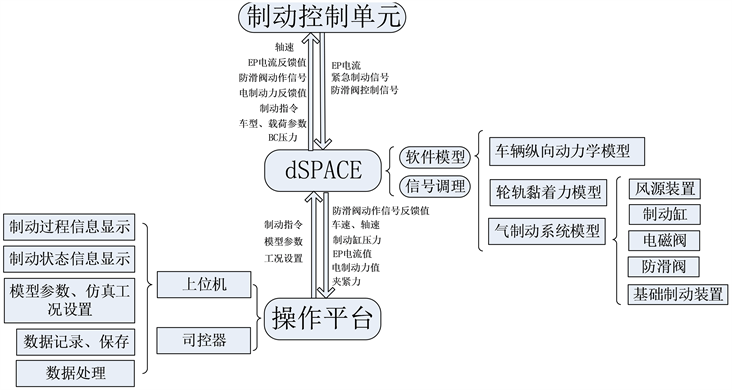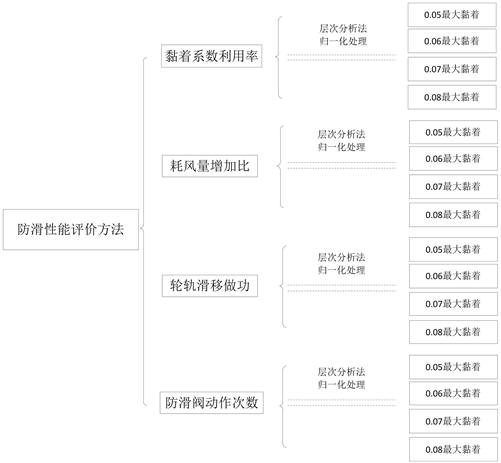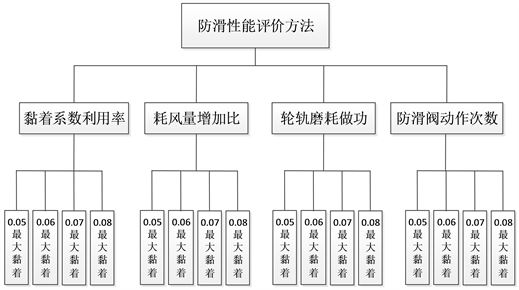# 基于层次分析法的列车防滑控制评价方法Evaluation Method of Anti-Sliding Control Based on Analytic Hierarchy Process

• 全文下载: PDF(1349KB)    PP.371-380   DOI: 10.12677/OJTT.2018.76045
• 下载量: 193  浏览量: 267   国家自然科学基金支持

Based on the HIL simulation platform and the research of existing WSP system evaluation, five design principles of WSP system performance evaluation method, including synthesis, comprehensiveness, adaptability, compulsion and repeatability, are put forward. Based on those principles, multi-index performance evaluation method of WSP system under different operating conditions as well as the standardization process of data processing is established.

1. 引言

2. 硬件在环半实物仿真平台Figure 1. HIL simulation platform framework for anti-skid control

1) 列车制动距离试验值为733米，制动距离仿真值为748米，误差约为2%，满足对试验中制动距离模拟的相关要求；

2) 制动过程中任一时刻仿真车速与试验车速最大差值为1.06 km/h，满足要求；

3) 各轴轴速仿真值与试验值对比分析结果见表1，其中第3轴防滑判据较为特殊，不同于其他三根轴，在此不作分析。速度差均值统计误差最大为2.91%，标准差统计误差最大为4.81%，模型性能满足要求。Table 1. Statistical comparison between simulation values and experimental values

3. 评价方法设计原则

1) 综合性原则

2) 全面性原则

3) 适应性原则

4) 强制性原则

5) 可重复性原则

4. 防滑控制系统性能评价方法建立

4.1. 防滑系统性能评价指标设计

4.1.1. 黏着系数利用率指标Figure 2. Schematic diagram of performance evaluation method for WSP systemFigure 3. Data acquisition process for evaluation method

$\eta =\frac{{s}_{\mathrm{min}}}{{s}_{real}}×100%$ (1)

4.1.2. 耗风量增加比指标

$V=\int Q\text{d}t$ (2)

$k\text{=}\frac{{V}_{滑}}{{V}_{干}}$ (3)

4.1.3. 轮对滑移做功指标

$P=\mu \cdot T\cdot \Delta v$ (4)

$W=\int \mu \cdot T\cdot \Delta v\text{d}t$ (5)

${W}_{avg}=\frac{\underset{1}{\overset{n}{\sum }}{W}_{i}}{n}\left(i=1,2,3,\cdots ,n\right)$ (6)

4.1.4. 防滑阀动作次数指标

${h}_{avg}=\frac{\underset{1}{\overset{4}{\sum }}{h}_{i}+\underset{1}{\overset{4}{\sum }}{r}_{j}}{8}$ (7)

4.2. 评价指标的归一化处理

${x}^{*}=\frac{x-{x}_{\mathrm{min}}}{{x}_{\mathrm{max}}-{x}_{\mathrm{min}}},{x}^{*}=1-\frac{x-{x}_{\mathrm{min}}}{{x}_{\mathrm{max}}-{x}_{\mathrm{min}}}$ (8)

${a}_{1}=\frac{\eta -0}{100%-0}$ (9)

${a}_{2}=1-\frac{k-1}{25-1}$ (10)

${a}_{3}=1-\frac{w-0}{26000-0}$ (11)

${a}_{4}=1-\frac{{h}_{avg}-0}{300-0}$ (12)

4.3. 基于层次分析法的各黏着水平指标值权重确立Table 2. Judgement matrix scale and its meaningFigure 4. Hierarchical structure establishment

${a}_{ij}={a}_{ik}/{a}_{jk};i,j,k=1,2,3,\cdots ,n$

1) 计算判断矩阵每一行元素的积，其中 $n$ 为矩阵阶数：

${M}_{i}=\underset{j=1}{\overset{n}{\prod }}{b}_{ij},i=1,2,3\cdots n$ (13)

2) 计算各行的 $n$ 次方根值： ${\stackrel{¯}{w}}_{i}=\sqrt[n]{{M}_{i}},i=1,2,3...\cdots n$

3) 将向量 ${\left({\stackrel{¯}{M}}_{1},{\stackrel{¯}{M}}_{2},{\stackrel{¯}{M}}_{3},\cdots .{\stackrel{¯}{M}}_{n}\right)}^{T}$ 归一化，计算如下，即为所求各指标的权重系数：

${w}_{i}=\frac{{\stackrel{¯}{w}}_{i}}{\underset{j=1}{\overset{n}{\sum }}{\stackrel{¯}{w}}_{j}}$ (14)

4) 判断矩阵的最大特征根为：

${\lambda }_{\mathrm{max}}=\underset{i=1}{\overset{n}{\sum }}\frac{{\left(AW\right)}_{i}}{n{w}_{i}}$ (15)Table 3. Mean random consistency index RI

5. 结束语

1) 基于综合性、全面性、适应性、强制性、可重复性等原则，从列车制动全过程黏着系数利用率、耗风量扩大比、轮对滑移做功、防滑阀动作次数等四个角度建立列车防滑系统评价方法；

2) 从多种轮轨黏着水平下对其性能进行考虑，使用min-max离差标准化及层次分析法对各指标进行归一化和标准化处理；

3) 确立了各性能指标所需采集量、计算方法以及数据归一化过程中各参数值的选取；

4) 完成层次分析法判断矩阵的建立及各指标权重的计算和一致性校验。

  邬志伟, 胡用生, 沈钢, 朱小娟. 城市轨道车辆牵引、制动与防滑系统的效率研究[J]. 城市轨道交通研究, 1998, 1(2): 32-36.  陈伟, 周军, 王新海, 曹宏发, 韩晓辉. 和谐号动车组制动防滑控制理论和试验.[J]. 铁道机车车辆, 2011, 31(5): 32-37.  李云峰. 城轨车辆空气制动防滑控制方法[J]. 铁道车辆, 2011, 49(12): 38-40+6.  Pugi, L., Malvezzi, M., Tarasconi, A., Palazzolo, A., Cocci, G. and Violani, M. (2006) Hil Simulation of WSP Systems on Mi-6 Test Rig. Vehicle System Dynamics, 44, 843-852.  Conti, R., Meli, E., Ridolfi, A. and Rindi, A. (2014) An Innovative Hardware in the Loop Architecture for the Analysis of Railway Braking under Degraded Adhesion Conditions through Roller-Rigs. Mechatronics, 24, 139-150. https://doi.org/10.1016/j.mechatronics.2013.12.011  Allotta, B., Meli, E., Ridolfi, A. and Rindi, A. (2014) Development of an Innovative Wheel-Rail Contact Model for the Analysis of Degraded Adhesion in Railway Systems. Tribology International, 69, 128-140. https://doi.org/10.1016/j.triboint.2013.09.013  Kang, C.-G., Kim, H.-Y., Kim, M.-S. and Goo, B.-C. (2009) Real-Time Simulations of a Railroad Brake System Using a dSPACE Board. IEEE ICCAS-SICE, Fukuoka, 18-21 August 2009.  Kang, C.-G. (2007) Analysis of the Braking System of the Korean High-Speed Train Using Real-Time Simulation. Journal of Mechanical Science and Technology, 21, 1048-1057. https://doi.org/10.1007/BF03027654  曹宏发, 周军, 陈伟. 基于仿真技术的防滑试验方法研究[J]. 铁道机车车辆, 2015, 35(3): 11-16.  Polach, O. (2005) Creep Forces in Simulation of Traction Vehicle Running of Adhesion Limit. Wear, 258, 992-1000. https://doi.org/10.1016/j.wear.2004.03.046  European Committee for Standardization (2009) BS EN 15595. Railway Applications Braking Wheel Slide Protection. CEN Management Centre, London.  吴云鼎, 解保生, 丁仲鹃. 实时定量PCR的数据归一化方法[J]. 昆明医科大学学报, 2013, 34(3): 160-164.  汤荣志, 段会用, 孙海涛. SVM训练数据归一化研究[J]. 山东师范大学学报(自然科学版), 2016(4): 60-65.  王丽. 基于AHP的城市旅游竞争力评价指标体系的构建及应用研究[J]. 地域研究与开发, 2014, 33(4): 105-108.  田辉平. 基于层次分析法和聚类分析法相结合的评价方法[J]. 华东经济管理, 2007(8): 126-128.